NCERT Solutions for Class 8 Maths Chapter 9 Algebraic Expressions and Identities Ex 9.1 are part of NCERT Solutions for Class 8 Maths. Here we have given NCERT Solutions for Class 8 Maths Chapter 9 Algebraic Expressions and Identities Ex 9.1.

 Board CBSE Textbook NCERT Class Class 8 Subject Maths Chapter Chapter 9 Chapter Name Algebraic Expressions and Identities Exercise Ex 9.1 Number of Questions Solved 4 Category NCERT Solutions

## NCERT Solutions for Class 8 Maths Chapter 9 Algebraic Expressions and Identities Ex 9.1

Question 1.
Identify the terms, their coefficients for each of the following expressions:
(i) $${ 5xyz }^{ 2 }-3zy$$
(ii) $$1+x+{ x }^{ 2 }$$
(iii) $$4{ x }^{ 2 }{ y }^{ 2 }-4{ x }^{ 2 }{ y }^{ 2 }{ z }^{ 2 }+{ z }^{ 2 }$$
(iv) 3 – pq + qr – rp
(v) $$\frac { x }{ 2 } +\frac { y }{ 2 } -xy$$
(vi) 0.3a – 0.6ab + 0.5b.
Solution.
(i) $${ 5xyz }^{ 2 }-3zy$$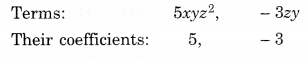(ii) $$1+x+{ x }^{ 2 }$$(iii) $$4{ x }^{ 2 }{ y }^{ 2 }-4{ x }^{ 2 }{ y }^{ 2 }{ z }^{ 2 }+{ z }^{ 2 }$$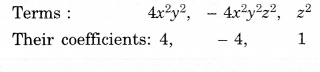(iv) 3 – pq + qr – rp(v) $$\frac { x }{ 2 } +\frac { y }{ 2 } -xy$$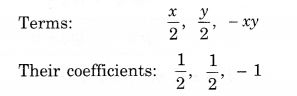(vi)0.3a – 0.6ab + 0.5b.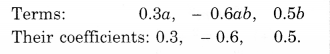Question 2.
Classify the following polynomials as monomials, binomials, trinomials. Which polynomials do not fit in any of these three categories?Solution.Question 3.
(i) ab – be, be – ca, ca – ab
(ii) a -b + ab, b – c + be, c – a + ac
(iii) $$2{ p }^{ 2 }{ q }^{ 2 }-3pq+4,\quad 5+7pq-3{ p }^{ 2 }{ q }^{ 2 }$$
(iv) $${ l }^{ 2 }+{ m }^{ 2 },\quad { m }^{ 2 }+{ n }^{ 2 },\quad { n }^{ 2 }+{ l }^{ 2 }$$, 2lm + 2mn + 2nl.
Solution.
(i) ab – be, be – ca, ca – ab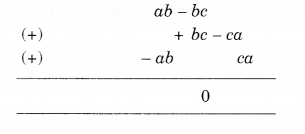(ii) a -b + ab, b – c + be, c – a + ac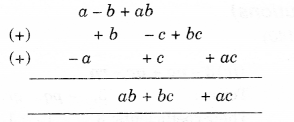(iii) $$2{ p }^{ 2 }{ q }^{ 2 }-3pq+4,\quad 5+7pq-3{ p }^{ 2 }{ q }^{ 2 }$$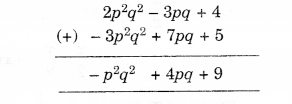(iv) $${ l }^{ 2 }+{ m }^{ 2 },\quad { m }^{ 2 }+{ n }^{ 2 },\quad { n }^{ 2 }+{ l }^{ 2 }$$, 2lm + 2mn + 2nl.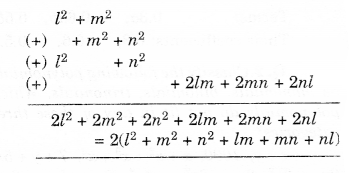Question 4.
(a) Subtract 4a – 7ab + 3b + 12 from 12a – 9ab + 56 – 3
(b) Subtract 3xy + 5yz – 7zx from 5xy – 2yz – 2zx + 10xyz
(c) Subtract $$4{ p }^{ 2 }q-3pq+5p{ q }^{ 2 }-8p+7q-10$$ from $$18-3p-11q+5pq-2p{ q }^{ 2 }+5{ p }^{ 2 }q$$
Solution.We hope the NCERT Solutions for Class 8 Maths Chapter 9 Algebraic Expressions and Identities Ex 9.1 help you. If you have any query regarding NCERT Solutions for Class 8 Maths Chapter 9 Algebraic Expressions and Identities Ex 9.1, drop a comment below and we will get back to you at the earliest.